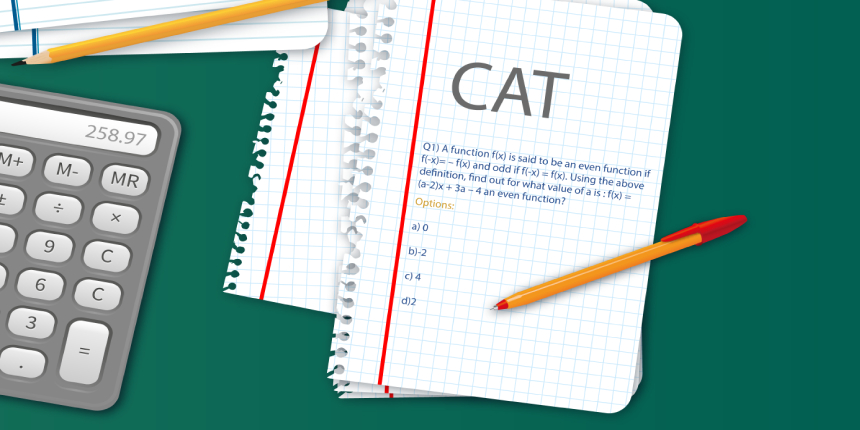Applications Open Now
SP Jain School of Global Management

CAT 2018 is just three weeks away and you must know how to deal with probably the toughest section in the exam - Logical Reasoning and Data Interpretation (LRDI). In this article, Dr. Shashank Prabhu who is a former CAT and IIFT topper with 100 percentile, tells how to crack the LRDI section. The tips will help the management aspirants who are appearing for CAT 2018 exam on November 25. The below article is brought to you by SDA Bocconi Asia Center and written by Dr, Shashank Prabhu to help aspirants in their test preparation. He had a scaled score of 249/360 in NMAT BY GMAC 2016 and 99 percentile in each of the sections and overall score. SDA Bocconi Asia Center accepts CAT, NMAT, GMAT, GRE for its International Master in Business.

Compare Colleges

College Comparison based on Courses, Placement, Rank, Fee

Applications Open Now
Ranked as the NO.1 Design institute| 99% Placement Record| 700+ Recruiters| Highest CTC: 8LPA

Logical reasoning and data interpretation are pretty fancy terms that are extremely ill-defined and so, it is not easy to prepare for the same keeping in mind a syllabus as one might do in case of verbal ability or quantitative ability.

There are two aspects to solving any reasoning based set: logic and retention. The sets are inherently easy to read and understand but the problem lies in representing the facts given in the form of usable data points. In case of simple question types like arrangements, grouping or even tables, this is easy to do by following classic norms. However, CAT has moved on from these elementary question types to questions that involve more ‘uncertainty’ and visualisation. The simplicity of the question makes sure that there is no better way of solving the question than simply forming the various cases one possibly can and infer the gameplay from there. With regard to the retention part, if you can build steps on top of each other while you are reading the set, it helps big time.

LRDI is not formulaic compared to the quantitative ability section and so, you have to think after each step. So, the better you are at plotting a roadmap from where you are to where you want to be, the easier would it be to crack the section. Practising interesting logical puzzles like Sudoku, Kakuro (which has had application in a lot of sets), Hitori, Bulls and Cows will improve your retention and help you connect the dots more easily. For basic practice, solving as many LRDI sets from previous year CAT and XAT papers is essential. In addition to this, mocks would help you understand the selection bit: which sets to prioritise and which ones to leave.

Here are some of the sets of LRDI from previous years’ CAT question paper.

Set 1

There were seven elective courses – E1 to E7 - running in a specific term in a college. Each of the 300 students enrolled had chosen just one elective from among these seven. However, before the start of the term, E7 was withdrawn as the instructor concerned had left the college. The students who had opted for E7 were allowed to join any of the remaining electives. Also, the students who had chosen other electives were given one chance to change their choice. The table below captures the movement of the students from one elective to another during this process. Movement from one elective to the same elective simply means no movement. Some numbers in the table got accidentally erased; however, it is known that these were either 0 or 1.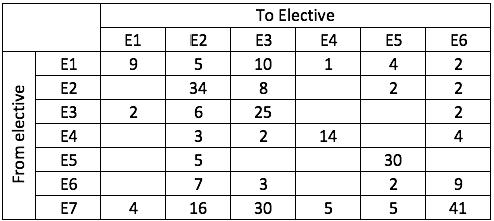Further, the following are known:

1. Before the change process there were 6 more students in E1 than in E4, but after the reshuffle, the number of students in E4 was 3 more than that in E1

2. The number of students in E2 increased by 30 after the change process

3. Before the change process, E4 had 2 more students than E6, while E2 had 10 more students than E3

Before we jump to the solution, the most important thing to note here is that, there has been an internal shuffle and so the number of students has remained the same. Also, the sum of all the numbers in a row signifies the number of people who were previously a part of the elective and the sum of all the numbers in a column signifies the number of people who are now a part of the elective. So, we will need to add the sum of rows and sum of columns when we are drawing the table on our rough sheet.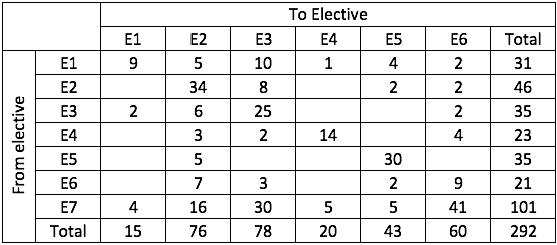Now, if we add all the numbers in the total column or the total row, we get the answer are 292. As each blank has either a 0 or a 1 in it, we can understand that there are eight 1s to be filled in the twelve blanks that exist.

If we look at the first addendum, before the change process there were 6 more students in E1 than in E4. As we have seen, the sum of all the numbers in a row gives us the number of students in an elective before the shuffle. We can see that there were 31 students in E1 before the shuffle. So, the number of students in E4 would have been 25 before the change process. As there are 2 blanks in the E4 row, both of them should be 1 to make the total 25.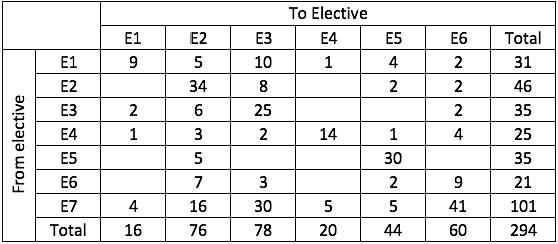We cannot do much about the after the shuffle data point in addendum 1. So, we move on to the next point.

The number of students in E2 increased by 30 after the change process. As we can observe, the total of the E2 column comes out to be 76. So, the sum of the numbers in the E2 row should be 46. Hence, the two blanks should both be equal to 0.

Now, the retention bit that I have spoken above comes into the picture. We had seen that there are twelve blanks and eight 1s. So, there ought to be four 0s hidden somewhere. We have just figured out two out of these four. Let’s look at the revised table: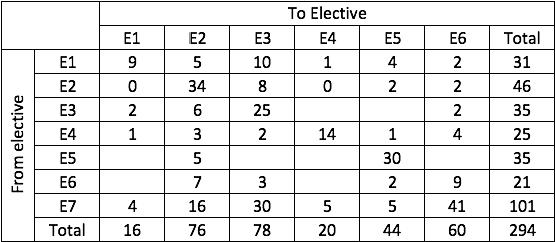In the third addendum, we have pre-shuffle data of E4, E6, E2 and E3. The best thing that can be done in this context is to look at the table and see which rows are filled. E2 and E4 have to be considered as our anchor cases in this situation because they have been filled completely.

The data says that before the change process, E4 had 2 more students than E6. So, E6 had 23 students before the change process. So, both the blanks should be 1s.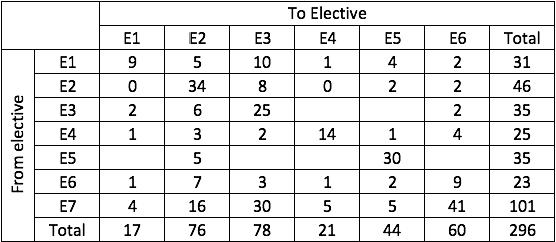The data also says that E2 had 10 more students than E3 before the change process. As we can see that E2 had 46 students before the shuffle, E3 would have had 36 students before the shuffle. So, one of the two blanks had to be 0 and the other had to be 1. If you remember, we had two more 0s to account for. We have taken care of one of them.

Now, there was a bit of information that we hadn’t used till now: after the reshuffle, the number of students in E4 was 3 more than that in E1. Let’s see if we can make sense of this.

If we look at the latest table that we have formed, the total of the numbers in the E1 column can be either 17 or 18. So, the total of the numbers in the E4 column would be either 20 or 21. As the sum of the existing numbers in the E4 column is already 21, the sum of numbers in the E1 column will have to be 18. So, the blank in the E1 column will be 1.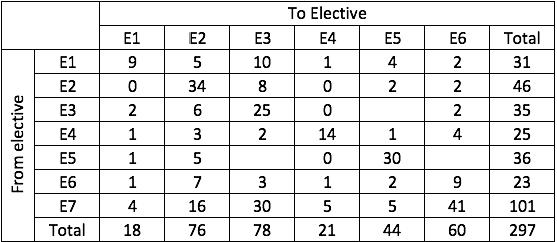Now, if you remember, we had two blanks in the E3 row before we did the previous step and we had figured out that one of these blanks had to be 0 and another had to be 1. As we have filled a 0 with this step, the other blank has to be 1.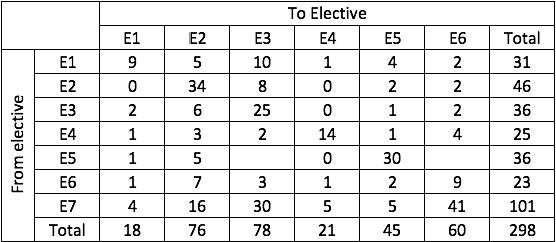Now, two blanks remain and two 1s have to be placed. So, we get the complete table as follows: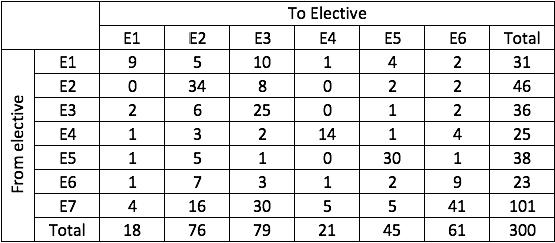Now, we can look at the questions and solve them.

Q1. How many elective courses among E1 to E6 had a decrease in their enrolments after the change process?

A. 4

B. 1

C. 2

D. 3

Here, we have to compare the row totals to the column totals and count the cases where the column total is less than the corresponding row total. We can see that it happens in two instances: E1 and E4. So, the answer is option C.

Q2. After the change process, which of the following is the correct sequence of number of students in the six electives E1 to E6?

A. 19, 76, 79, 21, 45, 60

B. 19, 76, 78, 22, 45, 60

C. 18, 76, 79, 23, 43, 61

D. 18, 76, 79, 21, 45, 61

As we can see, option D gives the correct sequence.

Q3. After the change process, which course among E1 to E6 had the largest change in its enrolment as a percentage of its original enrolment?

A. E1

B. E2

C. E3

D. E6

From our table, we can see that the change for E1, E2, E3, E4, E5 and E6 is 13, 30, 43, 4, 7 and 38 respectively. So, the answer is option C.

Q4. Later, the college imposed a condition that if after the change of electives, the enrolment in any elective (other than E7) dropped to less than 20 students, all the students who had left that course will be required to re-enrol for that elective. Which of the following is a correct sequence of electives in decreasing order of their final enrolments?

A. E2, E3, E6, E5, E1, E4

B. E3, E2, E6, E5, E4, E1

C. E2, E5, E3, E1, E4, E6

D. E2, E3, E5, E6, E1, E3

We can see that only E1 is less than 20 and so, there has to be a reversal. The calculation is pretty straightforward and we get the number of students in E1, E2, E3, E4, E5 and E6 as 31, 71, 69, 20, 41 and 59 respectively (simply subtract the number in the topmost row from the column total at the bottom). So, the decreasing order will be E2, E3, E6, E5, E1 and E4. Option 1 fits best.

The set was probably one of the more tedious ones in the section and a lot of people left it in pursuit of shorter, more intense sets. Considering that the only difficulty while solving this set was to make a table, we can now see why CAT LRDI is not as difficult as it is made out to be.

Applications Open NowUPES - School of Business MBABennett University MBAGraphic Era Hill University M...Ramaiah University of Applied...